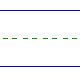Name: ______________________________________________ Date: _____________

Subtraction

Instructions: Subtract.

 5 - 2 =1 - 1 =4 - 2 =3 - 0 =4 - 3 =4 - 4 =3 - 2 =5 - 4 =1 - 0 =5 - 0 =4 - 1 =2 - 1 =3 - 3 =5 - 3 =3 - 1 =0 - 0 =5 - 5 =4 - 0 =